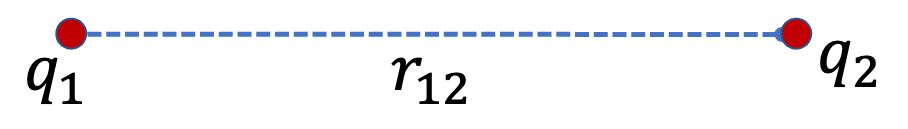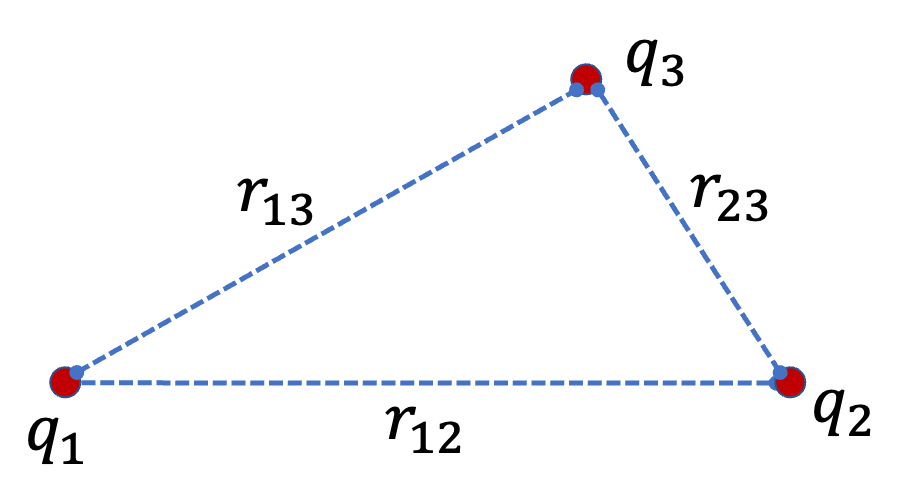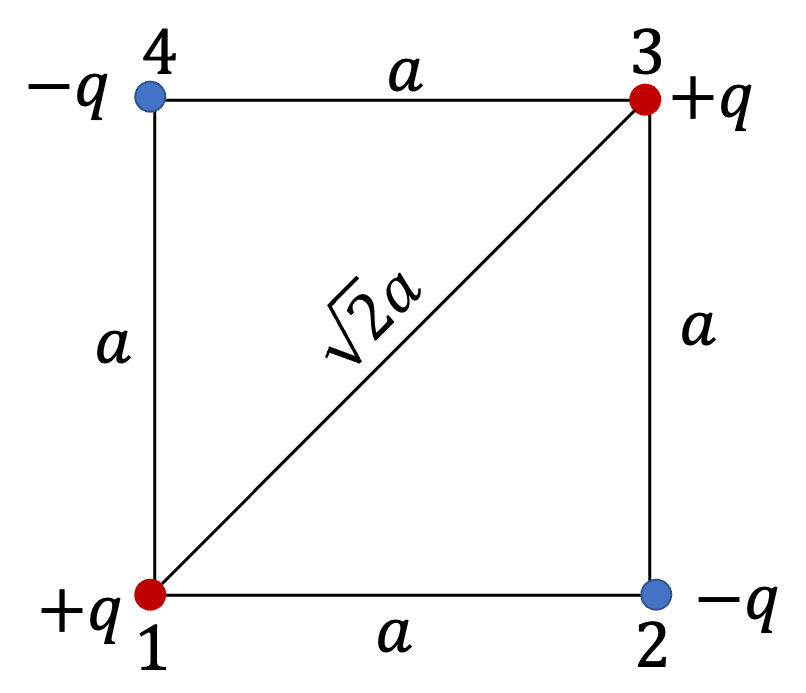## Section31.5Electrostatic Energy

Work necessary to assemble a charge collection from charges at infinitely separated configuration is called electrostatic energy of the collection. Any collection of charges can be built by bringing one charge at a time at the final location as following examples illustrate.

Assembling two charges $q_1$ and $q_2$ separated by a distance $r_{12}\text{.}$

We first bring charge $q_1$ from infinity and fix it at the origin. Since there was no charge before this charge, we would need no work to accomplish this since there is no force on the charge.

\begin{equation*} W_1 = 0 \end{equation*}

Next, we bring $q_2$ from infinity to a point that is a distance $r_{12}$ from $q_1\text{.}$The work done by the agent for bringing this charge will equal the change in the potentialenergy of $q_2\text{.}$ That is,

\begin{equation*} W_2 = q_2\,\phi_1 = \dfrac{1}{4\pi\epsilon_0}\, \dfrac{q_1 q_2}{r_{12}}. \end{equation*}

Adding $W_1$ and $W_2$we get the net work of assembling the charge distribution. Thus, electrostatic energy of the two-charge system will be

\begin{equation*} U = W_1 + W_2 = \dfrac{1}{4\pi\epsilon_0}\, \dfrac{q_1 q_2}{r_{12}}. \end{equation*}

Assembling three charges $q_1\text{,}$ $q_2\text{,}$ and $q_3$ separated by distance $r_{12}\text{,}$ $r_{13}\text{,}$ and $r_{23}$

We assemble this structure one charge at a time. We already know how much work it will cost to assemble the first two charges.

\begin{align*} W_1 \amp = 0,\\ W_1 \amp = \dfrac{1}{4\pi\epsilon_0}\, \dfrac{q_1 q_2}{r_{12}}. \end{align*}Now, we hold $q_1$ and $q_2$ fixed it their final positions, and bring $q_3$ fron infinity in the common field of both $q_1$ and $q_2\text{.}$ The work will be equal to the change in potential energy of $q_3\text{,}$ which will be

\begin{equation*} W_3 = q_3 \times \left( \phi_{13} + \phi_{23} \right), \end{equation*}

where $\phi_{13}$ is the potential of $q_1$ at the final location of $q_3$ and similarly for $\phi_{23}\text{.}$ Therefore,

\begin{equation*} W_3 = \dfrac{1}{4\pi\epsilon_0}\, \dfrac{q_1 q_3}{r_{13}} + \dfrac{1}{4\pi\epsilon_0}\, \dfrac{q_2 q_3}{r_{23}}. \end{equation*}

Now, we add up all the three work for the three-particle system.

\begin{align} U \amp = W_1 + W_2 + W_3\notag\\ \amp = \sum_{\lt ij \gt \text{ pairs}}\ \dfrac{1}{4\pi\epsilon_0}\, \dfrac{q_i q_j}{r_{ij}}. \label{eq-electrostatic-energy-3-charges}\tag{31.5.1} \end{align}

Assembling $N$ charges $q_1\text{,}$ $q_2\text{,}$ $\cdots\text{,}$ $q_N$ separated by distance $r_{12}\text{,}$ $r_{13}\text{,}$ $\cdots$

Eq. (31.5.1) shows a general way to think about the problem. We will get energy from every pair of charges. We need to be careful to count each pair only once.

\begin{equation} U = \sum_{\lt ij \gt \text{ pairs}}\ \dfrac{1}{4\pi\epsilon_0}\, \dfrac{q_i q_j}{r_{ij}}.\label{eq-electrostatic-energy-N-charges}\tag{31.5.2} \end{equation}

### Subsection31.5.1(Calculus) Electrostatic Energy of a Continuous Charge System

Equation (31.5.2) can be generaized for application to continuous charges. First we note that we can count each pair double and divide by 2 to get the same answer. We can treat the sum as two sums, one for $i$ and the other for $j\text{.}$ Factor out $q_i\text{,}$ we find that the other sum is just potential of all other charges at the location of $i\text{.}$

\begin{align*} U \amp = \dfrac{1}{2} \sum_{i} q_i \left[\sum_{j\ne i}\ \dfrac{1}{4\pi\epsilon_0}\, \dfrac{ q_j}{r_{ij}} \right].\\ \amp = \dfrac{1}{2} \sum_{i} q_i\, \phi_{i}, \end{align*}

where $\phi_i$ is the potential of other charges at the location of charge $i\text{.}$ Treating this for continuous charges is simply replacing $q_i$ by $\rho(x,y,z)dV\text{,}$ $\phi_{\text{i}$ by $\phi(x,y,z)\text{,}$ and the sum by integral over entire space.

\begin{equation} U = \dfrac{1}{2}\iiint \rho(x,y,z)\, \phi(x,y,z)\, dV.\label{eq-electrostatic-energy-continuous-charges}\tag{31.5.3} \end{equation}

Four charges, $+q,\ -q,\ +q,\ -q$ alternate on corners of a square of side $a\text{.}$ What is the electrostatic energy?

Hint

Adjacent pairs will be negative energy and diagonals will be positive energy.

$-\dfrac{1}{4\pi\epsilon_0}\; \dfrac{q^2}{a}(4-\sqrt{2})\text{.}$

Solution

Electrostatic energy has contribution from every pair counted only once. We have pairs $(1,2)\text{,}$ $(1,3)\text{,}$ $(1,4)\text{,}$ $(2,3)\text{,}$ $(2,4)\text{,}$ $(3,4)\text{.}$ The pairs $(1,3)$ and $(2,4)$ have positive energy and the others have negative.\begin{align*} U \amp = \dfrac{q^2}{4\pi\epsilon_0}\left( -4\times \dfrac{1}{a} + 2\times \dfrac{1}{\sqrt{2}\; a} \right)\\ \amp= -\dfrac{1}{4\pi\epsilon_0}\; \dfrac{q^2}{a}(4-\sqrt{2}). \end{align*}

To form a helium atom, an alpha particle which contains two protons and two neutrons was fixed at one place, and two electrons were brought from far away one at a time. The first electron was placed at $6.0 \times 10^{-11}\text{ m}$ from the alpha particle and held there while the second electron was brought to $6.0 \times 10^{-11}\text{ m}$ from the alpha particle on the other side of the first electron.

(a) How much work was done in each step in $\text{J}\text{?}$

(b) What is the electrostatic energy of the alpha particle and two electrons in the final configuration in $\text{J}\text{?}$

Hint

Work equal to the change in potential energy needs to be provided.

(a) $-7.68\times 10^{-18}\text{ J}\text{,}$ $-5.76\times 10^{-18}\text{ J}\text{;}$ (b) $-1.34\times 10^{-17}\text{ J}\text{.}$

Solution

In each step we need to provide energy equal to the change in potential energy of the incoming electron, which is brough from infinity, where energy is zero.

(a)

\begin{align*} W_1 \amp = k \dfrac{2e\times (-e)}{r}\\ \amp = -9\times 10^9 \times \dfrac{2\times \left(1.6\times 10^{-19}\right)^2}{0.6\times 10^{-10}}\\ \amp = -7.68\times 10^{-18}\:\text{J},\\ W_2 \amp = k \left[ \dfrac{2e\times (-e)}{r} + \dfrac{(-e)\times (-e)}{2r} \right] \\ \amp = \dfrac{3}{4}W_1 = -5.76\times 10^{-18}\:\text{J}. \end{align*}

Since work is negative, we will need to extract energy away from the system.

(b) The total work in assembling a charge configuration is called its electrostatic energy. Hence,

\begin{equation*} U = W_1 + W_2 = -1.34\times 10^{-17}\:\text{J}. \end{equation*}

Since work is negative, we will need to extract energy away from the system.

A sphere of radius $R$ is uniformly charged with total charge $q\text{.}$ What is the electrostatic energy?

Hint: Electric potential at a point inside the sphere = $\dfrac{1}{4\pi\epsilon_0} \dfrac{q}{R} \left( \dfrac{3}{2} - \dfrac{1}{2}\; \dfrac{r_{\text{in}}^2}{R^2} \right)\text{.}$ See Checkpoint 31.7.5.

Hint

Use the potential for inside points.

$\dfrac{\rho q R^2}{5\epsilon_0} \text{.}$
Since the potential and charge are spherical, the integral simplifies to integral over $r$ only with $dV = 4\pi r^2 dr$